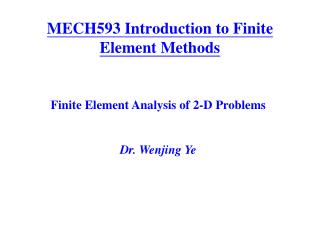Download PresentationMECH593 Introduction to Finite Element Methods

MECH593 Introduction to Finite Element Methods - PowerPoint PPT Presentation

MECH593 Introduction to Finite Element Methods. Finite Element Analysis of 2-D Problems Dr. Wenjing Ye. 2-D Discretization. Common 2-D elements: . 2-D Model Problem with Scalar Function - Heat Conduction. Governing Equation. in W. Boundary Conditions. Dirichlet BC: . Natural BC: .I am the owner, or an agent authorized to act on behalf of the owner, of the copyrighted work described.
Download PresentationMECH593 Introduction to Finite Element Methods

Download Policy: Content on the Website is provided to you AS IS for your information and personal use and may not be sold / licensed / shared on other websites without getting consent from its author.While downloading, if for some reason you are not able to download a presentation, the publisher may have deleted the file from their server.

- - - - - - - - - - - - - - - - - - - - - - - - - - E N D - - - - - - - - - - - - - - - - - - - - - - - - - -
Presentation Transcript
1. MECH593 Introduction to Finite Element Methods Finite Element Analysis of 2-D Problems Dr. Wenjing Ye

2. 2-D Discretization Common 2-D elements:

3. 2-D Model Problem with Scalar Function- Heat Conduction • Governing Equation in W • Boundary Conditions Dirichlet BC: Natural BC: Mixed BC:

4. Weak Formulation of 2-D Model Problem • Weighted - Integralof 2-D Problem ----- • Weak Form from Integration-by-Parts-----

5. Weak Formulation of 2-D Model Problem • Green-Gauss Theorem ----- where nx and ny are the components of a unit vector, which is normal to the boundary G.

6. Weak Formulation of 2-D Model Problem • Weak Formof 2-D Model Problem ----- EBC: Specify T(x,y) on G NBC: Specify on G where is the normal outward flux on the boundary G at the segment ds.

7. FEM Implementation of 2-D Heat Conduction – Shape Functions Step 1: Discretization – linear triangular element (T3) T1 Derivation of linear triangular shape functions: T2 Let T3 Interpolation properties Same

8. FEM Implementation of 2-D Heat Conduction – Shape Functions f3 f2 f1 linear triangular element – local (area) coordinates T1 A2 A3 A1 T2 T3 T1 T2 T3

9. FEM Implementation of 2-D Heat Conduction – Shape Functions quadratic triangular element (T6) – local (area) coordinates T1 T4 T6 T2 T3 T5 T1 T4 T6 T2 T3 T5 Serendipity Family – nodes are placed on the boundary for triangular elements, incomplete beyond quadratic

10. Interpolation Function - Requirements • Interpolation condition • Take a unit value at node i, and is zero at all other nodes • Local support condition • fiis zero at an edge that doesn’t contain node i. • Interelement compatibility condition • Satisfies continuity condition between adjacent elements over any element boundary that includes node i • Completeness condition • The interpolation is able to represent exactly any displacement field which is polynomial in x and y with the order of the interpolation function

11. Formulation of 2-D 4-Node Rectangular Element – Bi-linear Element (Q4) Let h 4 3 x 1 2 Note: The local node numbers should be arranged in a counter-clockwise sense. Otherwise, the area Of the element would be negative and the stiffness matrix can not be formed. f2 f4 f1 f3

12. Formulation of 2-D 4-Node Rectangular Element – Bi-linear Element (Q4) Reference domain (master element) Physical domain (physical element) h h 3 4 3 4 x x y 1 1 2 2 x

13. FEM Implementation of 2-D Heat Conduction – Element Equation • Weak Formof 2-D Model Problem ----- Assume approximation: and let w(x,y)=fi(x,y) as before, then where

14. Linear Triangular Element Equation where is the length vector from the ith node to the jth node.

15. Imposing Boundary Conditions The meaning of qi: 3 3 1 1 1 2 2 3 3 1 1 1 2 2

16. Imposing Boundary Conditions Consider Equilibrium of flux: FEM implementation:

17. Calculating the q Vector Example:

18. 2-D Steady-State Heat Conduction - Example A D AB: CD: convection DA and BC: 0.6 m C B 0.4 m y x